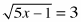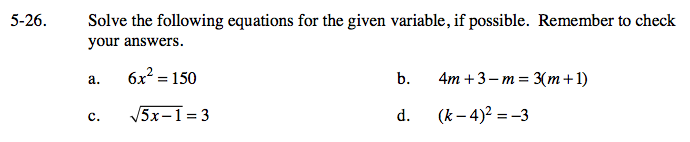### Home > GC > Chapter 5 > Lesson 5.1.3 > Problem5-26

5-26.
1. Solve the following equations for the given variable, if possible. Remember to check your answers. Homework Help ✎

1. 6x2 = 150

2. 4m + 3 − m = 3(m + 1)

3.4. (k − 4)2 = −3Isolate the x². There are two solutions to this equation.

All numbers, because the expressions on both sides are the same 3m + 3 = 3m + 3

Start by squaring both sides.

No real numbers, because any number squared is a positive number, so (k − 4)² ≠ −3.